# Model Tuning in Machine Learning

## Model Tuning Techniques in Machine Learning

In this article, I am going to discuss Model Tuning Techniques in Machine Learning with Examples. Please read our previous article where we discussed ETS Models in Machine Learning with Examples. At the end of this article, you will understand the different Model Tuning Techniques in Machine Learning.

##### Introduction to Model Tuning in Machine Learning

Before we understand model tuning in more depth, let’s get to know what are parameters and hyperparameters?

##### What are the Parameters?

Internal variables that can be approximated from the training data are known as parameters. They alter during training and are retained as part of the learned model afterward. The model makes predictions based on parameters. The characteristics of parameters are –

1. These are trained
2. These are internal values of a model
3. These are estimated/altered by learning from data
4. These values are stored as a part of the trained model
##### What are Hyperparameters?

Hyperparameters are user-defined external configurations. They don’t alter while the training task is running and govern or impact how the model learns throughout training. The goal of model tuning is to optimize the values of Hyperparameters. Major characteristics of hyperparameters are –

1. These are tuned
2. They are external values
3. These are defined by a user
4. These aren’t part of a trained model.

Hyperparameter optimization is another term for model tuning. The training process is controlled by hyperparameters, which are variables. During a model training job, these are configuration variables that do not change. Model tweaking gives ideal values for hyperparameters, increasing the predicted accuracy of your model.

Each model has its own set of Hyperparameters, some of which are unique to it and others that are shared by a group of algorithms. Tree depth and maximum leaf nodes, for example, are hyperparameters in XG boost, whereas the number of layers and hidden width are hyperparameters in Neural Networks.

When adjusting hyperparameters to check if the model improves, keep the following in mind:

1. Which hyperparameters have the greatest impact on your model?
2. Which values should you choose?
3. How many hyperparameter combinations should you try?

The behavior of the modeling algorithm is controlled via hyperparameters. During the initialization of the algorithm, hyperparameters are provided as inputs. (For example, specifying a criterion for decision tree construction.)

The function get_params() can be used to find out about the hyperparameters for an algorithm.

##### How are Hyperparameters Fine-Tuned?
1. The type of model, whether it’s a classifier or a regressor, must be chosen.
2. Keep an eye out for the parameter space.
3. Select a sample method for the parameter space.
4. To ensure that the model can generalize, use the cross-validation approach.

Methodology for searching hyperparameter space –

1. GridSearchCV – It combines all of the parameters.
2. RandomizedSearchCV – It can sample a specified number of possibilities from a parameter space with a given distribution.

To avoid data leakage, the data should always be separated into three stages during hyper-parameter tuning: training, validation, and testing.

To convert the test data individually, use the same set of functions that were used to alter the rest of the data for creating models and hyperparameter tuning.

##### Parameter Tuning using GridSearchCV

Now that we understand what hyperparameters are, our goal should be to identify the ideal hyperparameter values in order to obtain the best prediction results from our model. However, the question of how to identify the best sets of hyperparameters arises. The Manual Search method can be used to determine the optimal hyperparameters by utilizing a hit-and-miss approach, which would take a long time to develop a single model.

As a result, technologies like Random Search and GridSearch were developed. In this section, we’ll go over how Grid Search works and how GridSearchCV handles cross-validation.

Grid Search calculates the performance for each combination of all the supplied hyperparameters and their values and then chooses the optimum value for the hyperparameters. Based on the amount of hyperparameters involved, this makes the processing time-consuming and costly.

GridSearchCV does cross-validation in addition to grid search. The model is trained using cross-validation. As we all know, we divide the data into two pieces before training the model with it: train data and test data. The method of cross-validation divides the train data into two parts: the train data and the validation data.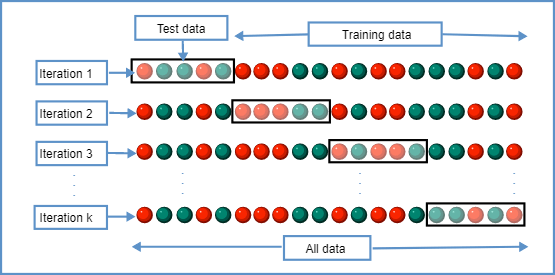K-fold Cross-validation is the most common type of cross-validation. The training data is divided into k divisions using an iterative approach. One division is kept for testing and the remaining k-1 partitions are used to train the model in each iteration.

The scikit-learn class model selection has a GridSearchCV() method. It can be started by constructing a GridSearchCV() object:

classifier = GridSearchCV(estimator, param_grid, cv, scoring)

It requires four arguments: estimator, param grid, cv, and scoring. The following is a list of the arguments:

1. estimator – a model built with Scikit-learn
2. param_grid – A dictionary containing lists of parameter values and parameter names as keys.
3. scoring – the criterion for evaluating performance. For example, for regression models, ‘r2’ is used, while for classification models, ‘precision’ is used.
4. cv – The number of folds for K-fold cross-validation is an integer.

GridSearchCV can be used on many hyperparameters to find the optimum values for the hyperparameters that are supplied.

Let’s take a look at this example for the implementation of hyperparameter tuning using grid search –

```# Import Necessary Libraries
import pandas as pd
from sklearn.ensemble import RandomForestClassifier
from sklearn.model_selection import train_test_split
from sklearn.model_selection import GridSearchCV
from sklearn import metrics

# Import Dataset
df = pd.DataFrame(data=data.data, columns=data.feature_names)
df['target'] = pd.Series(data.target)
```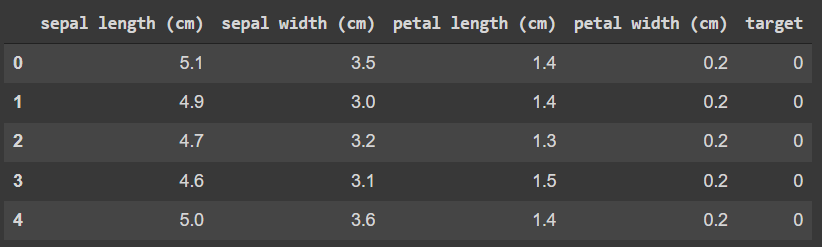```# Train Test Data Split
X = df.drop('target', axis = 1)
y = df['target']

X_train, X_test, y_train, y_test = train_test_split(X, y, test_size = 0.2, random_state = 42)

# Model Building
classifier = RandomForestClassifier()

# Hyper parameter Tuning
forest_params = [{'max_depth': list(range(10, 15)), 'max_features': list(range(0,14))}]
model = GridSearchCV(classifier, forest_params, cv = 10, scoring='accuracy')
model.fit(X_train, y_train)
print(model.best_params_)
print(model.best_score_)
```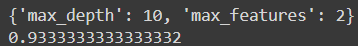The optimum combination of tweaked hyperparameters is given by the model.best_params_, while the average cross-validated score of our Random Forest Classifier is given by the model.best_score_.

##### Parameter Tuning using Random Search in Machine Learning

We construct distributions for each hyperparameter in a random search, which can be defined uniformly or via a sampling method. The main distinction between random search and grid search is that with random search, not all of the values are examined, and the values that are tested are chosen at random.

For example, if the distribution has 500 values and we specify n iter=50, a random search will randomly select 50 of them to evaluate. By doing so, the random search saves time while also allowing it to investigate additional values in the specified distribution without having to define an absolute grid.

Because random search does not test every hyperparameter combination, it does not always return the highest performing values, but it does return a model that performs reasonably well in a shorter amount of time.

The scikit-learn class model selection has a RandomizedSearchCV() method. It can be started by constructing a RandomizedSearchCV() object:

classifier = RandomizedSearchCV(estimator, param_distributions, scoring)

It requires four arguments: estimator, param grid, cv, and scoring. The following is a list of the arguments:

1. estimator – a model built with Scikit-learn
2. param_distributions – A dictionary containing lists of parameter values and parameter names as keys.
3. scoring – the criterion for evaluating performance. For example, for regression models, ‘r2’ is used, while for classification models, ‘precision’ is used.

Let’s take a look at this example for the implementation of hyperparameter tuning using grid search –

```# Import Necessary Libraries
import pandas as pd
from sklearn.ensemble import RandomForestClassifier
from sklearn.model_selection import train_test_split
from sklearn.model_selection import RandomizedSearchCV
from sklearn import metrics

# Import Dataset
df = pd.DataFrame(data=data.data, columns=data.feature_names)
df['target'] = pd.Series(data.target)
``````# Train Test Data Split
X = df.drop('target', axis = 1)
y = df['target']

X_train, X_test, y_train, y_test = train_test_split(X, y, test_size = 0.2, random_state = 42)

# Model Building
classifier = RandomForestClassifier()

# Hyperparameter Tuning
param_dist = {
'n_estimators': list(range(50, 300, 10)),
'min_samples_leaf': list(range(1, 50)),
'max_depth': list(range(2, 20)),
'max_features': ['auto', 'sqrt'],
'bootstrap': [True, False]}

model = RandomizedSearchCV(classifier, param_dist, scoring='accuracy')
model.fit(X_train, y_train)
print(model.best_params_)
print(model.best_score_)
```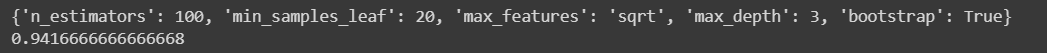##### Which ML Algorithm should you choose?

This is a generic, practical strategy for ML challenges, and it involves selecting an appropriate model based on the business problem and dataset:

##### 1. Sort the Issue into Categories –

Categorize by input: If the data is labeled, it’s a supervised learning problem. It’s an unsupervised learning problem if the data is unlabeled and the goal is to uncover structure. It’s a reinforcement learning problem if the answer entails optimizing an objective function by interacting with the environment.

Categorize by output: If the model’s output is a number, you’re dealing with a regression problem. It’s a classification problem if the model’s output is a class. It’s a clustering problem if the model’s output is a set of input groups.

##### 2. Analyze Your Data –

Data is the raw material for the entire analytic process, not the final product. Successful businesses not only collect and access data, but also use it to extract insights that help them make better decisions, which leads to improved customer service, competitive differentiation, and revenue development. The process of comprehending the data is critical to selecting the best algorithm for the job. Some algorithms can function with smaller sample sets, while others need a large number of them. Certain algorithms prefer to operate with categorical data, whereas others prefer numerical data.

Data Analysis – Understanding data with descriptive statistics and understanding data with visualization and plots are two crucial tasks at this level.

Data Preprocessing – Pre-processing, profiling, and cleansing are all aspects of data processing, and it frequently entails combining data from many internal and external systems.

Data Transformation – Feature engineering is the conventional idea of converting data from a raw state to a state appropriate for modeling. Transform data and feature engineering are often used interchangeably. Here’s how to define the latter term. The process of changing raw data into features that better describe the underlying problem to predictive models, resulting in enhanced model accuracy on unseen data, is known as feature engineering.

##### 3. Locate the Algorithms that are Available –

Following the classification of the problem and comprehension of the data, the following step is to select the algorithms that are suitable and realistic to apply in a fair amount of time. The following are some of the factors that influence model selection:

• The model’s accuracy.
• The model’s interpretability.
• The model’s complication.
• The model’s ability to scale.
• When it comes to building, training, and testing the model, how long does it take?
• How long does it take to use the model to make predictions?
• Is the model in line with the company’s objectives?
##### 4. ML Algos Implementation –

Create a machine learning pipeline that assesses each algorithm’s performance on the dataset against a set of carefully chosen assessment criteria. Another option is to apply the same technique to different dataset subgroups. The ideal solution is to do it once or to create a service that does it at regular intervals as new data is added.

If necessary, grid search, random search, and Bayesian optimization are the three methods for optimizing hyperparameters.

You can follow this flowchart to choose an algorithm accordingly –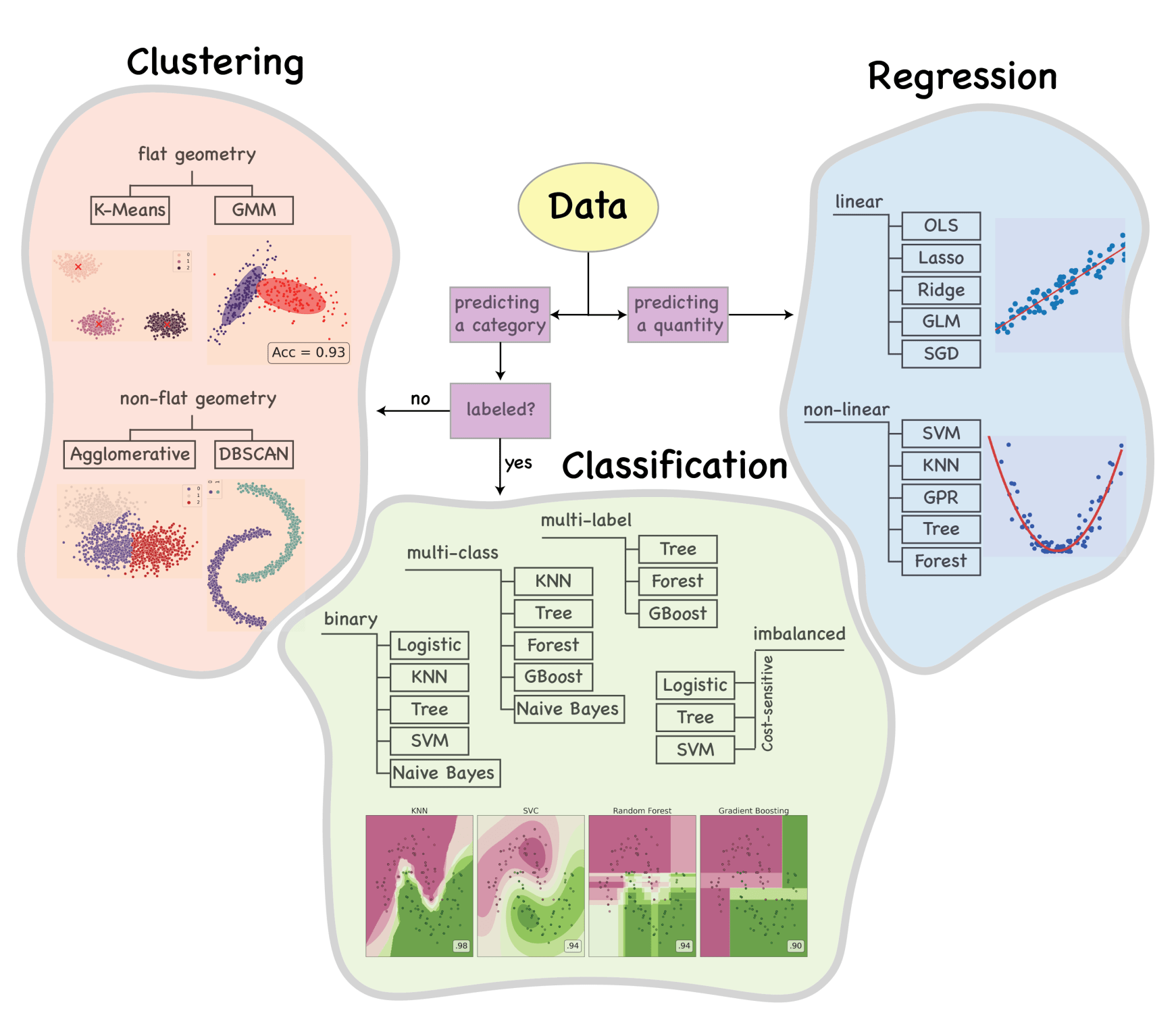##### How to Compare Machine Learning Algorithms in Practice?

Each model or machine learning algorithm has a number of features that handle data in various ways. The data provided to these algorithms are frequently changing depending on the stage of the experiment. However, because machine learning teams and developers typically document their experiments, there is plenty of data to compare.

The difficulty is determining which parameters, data, and information must be evaluated before making a final decision. It’s the classic paradox of having an abundance of information but no clarity.

Even more difficult, we must determine whether a high-value parameter, such as a higher metric score, indicates that the model is better than one with a lower score, or if it is simply due to statistical bias or misdirected metric design.

Comparing machine learning algorithms is vital in and of itself, but there are some less obvious advantages to efficiently comparing different studies. Let’s take a look at the comparison objectives:

##### 1. Enhanced performance

The fundamental goal of model comparison and selection is to improve the machine learning software/performance. The goal is to find the optimal algorithms that fit the data as well as the business needs.

##### 2. Longer Life Expectancy

If the chosen model is tightly associated with the training data and fails to understand unseen data, high performance may be short-lived. As a result, it’s also critical to build a model that comprehends underlying data patterns so that predictions stay a long time and retraining is low.

##### 3. Retraining is Less Difficult

When models are reviewed and prepared for comparison, small information and metadata are captured, which might be useful during retraining. If a developer can clearly retrace the reasons for selecting a model, for example, the causes of model failure will instantly become apparent, and retraining can begin at the same time.

##### 4. Production that is Quick

It’s simple to narrow down on models that can give fast processing speed and make the best use of memory resources with the model specifications at hand. Several factors are required to customize the machine learning solutions throughout the production as well. Having production-level data can help you align with the production engineers more readily. Furthermore, knowing the resource requirements of various algorithms will make it easier to ensure that they are compliant.

##### Machine learning algorithm parameters and how to compare them?

Let’s get started evaluating and comparing the many characteristics of algorithms that may be used to rank and select the best machine learning models. The comparable parameters have been split into two categories at a high level:

1. Based on development, and
2. Characteristics depending on production
##### Statistical Evaluations

Machine learning models, at their most basic level, are statistical equations that run at high speeds on a large number of data points to arrive at a result. As a result, performing statistical tests on the algorithms is crucial for both correcting them and determining whether the model’s equation is the best match for the dataset at hand. Here are a few common statistical tests that can be used to establish a baseline for comparison:

1. Null Hypothesis Test
2. ANOVA Test
3. Chi-Square Test
4. T-Test
5. K-fold Cross-Validation
##### Model Features

It’s critical to evaluate the model’s features or parameters when selecting the optimum machine learning model for a given dataset. The model’s flexibility, assumptions, and learning style may all be assessed using the parameters and model objectives.

##### Learning Curves

They can assist in determining whether or not a model is on the right learning path to achieve the bias-variance tradeoff. It also serves as a benchmark for evaluating different machine learning models: a model with consistent learning curves throughout both the training and validation sets is more likely to perform well on new data over time. Learning curves are the most effective approach to tracking model training progress. These curves aid in the selection and evaluation of models by identifying the best combinations of hyperparameters.

##### Loss Metrics and Functions

Loss functions and metric functions are frequently confused. Model optimization and tweaking are done with loss functions, while model evaluation and selection are done with metric functions. Due to the inability to measure regression accuracy, the same metrics are utilized to evaluate performance as well as model error for optimization.

Loss functions are supplied to models as parameters, allowing them to be modified to minimize the loss function. When the model makes an inaccurate judgment, the loss function imposes a severe penalty.

Evaluation Metrics for Classification ML problems –

1. Confusion Matrix
2. Accuracy
3. Precision and Recall
4. F1 Score
5. AUC-ROC
6. Log Loss

Evaluation Metrics for Regression ML problems –

1. MAE (Mean Absolute Error)
2. MSE (Mean Squared Error)
3. RMSE (Root Mean Squared Error)
4. R-Squared Error
##### Parameters Dependent on Production

Until now, we’ve seen similar model elements that are prioritized during the development phase. Let’s look at a few production-oriented features that can help you save time on production and processing.

##### Complexity of Time

Depending on the use case, the temporal complexity may be the most important factor to consider when selecting a model. For example, the K-NN classifier is best avoided for a real-time solution since it calculates the distance of new data points from the training points at the time of prediction, making it a sluggish method. A slow predictor, on the other hand, isn’t a huge deal for solutions that require batch processing.

Given the chosen model, the time complexities during the training and testing phases may change. For example, during training, a decision tree must estimate the decision points, however during prediction, the model must simply apply the conditions that are already present at the predetermined choice points. If the solution necessitates frequent retraining, such as in a time series solution, selecting a model with speed during both training and testing will be the best option.

##### Complexity of Space

To compare distances, the model must load the whole training data into memory every time it wants to forecast, as seen in the K-NN example above. If the training data is large, it might represent a costly drain on the company’s resources, such as RAM or storage space given for the solution. Processing and computation functions should always have enough memory in RAM. The speed and processing capacity of a solution can be harmed by loading an excessive volume of data.

In the next article, I am going to discuss Model Selection Techniques in Machine Learning with Examples. Here, in this article, I try to explain Model Tuning Techniques in Machine Learning with Examples. I hope you enjoy this Model Tuning Techniques in Machine Learning with Examples article. Please post your feedback, suggestions, and questions about this Model Tuning Techniques in Machine Learning article.